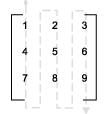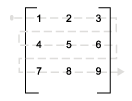## Code Generation of Matrices and Arrays

MATLAB® stores matrix data and arrays (1–D, 2–D, ...) in column-major format as a vector. Simulink® and the code generator can store array data in column-major or row-major format. For an array stored in column-major layout, the elements of the columns are contiguous in memory. In row-major layout, the elements of the rows are contiguous. Array layout is also referred to as order, format, and representation. The order in which elements are stored can be important for integration, usability, and performance. Certain algorithms perform better on data stored in a particular order.

Programming languages and environments assume a single array layout for all data. MATLAB and Fortran use column-major layout by default, whereas C and C++ use row-major layout. With Simulink Coder™, you can generate C/C++ code that uses row-major layout or column-major layout.

### Array Storage in Computer Memory

Computer memory stores data in terms of one-dimensional arrays. For example, when you declare a 3-by-3 matrix, the software stores this matrix as a one-dimensional array with nine elements. By default, MATLAB stores these elements with a column-major array layout. The elements of each column are contiguous in memory.

Consider the matrix `A`:

```A = 1 2 3 4 5 6 7 8 9```
`A` translates to an array of length 9 in this order:
```A(1) = A(1,1) = 1; A(2) = A(2,1) = 4; A(3) = A(3,1) = 7; A(4) = A(1,2) = 2; A(5) = A(2,2) = 5;```
and so on.

In column-major format, the next element of an array in memory is accessed by incrementing the first index of the array. For example, these element pairs are stored sequentially in memory:

• `A(i)` and `A(i+1)`

• `B(i,j)` and `B(i+1,j)`

• `C(i,j,k)` and `C(i+1,j,k)`

The matrix `A` is represented in memory by default with this arrangement:

` 1 4 7 2 5 8 3 6 9`

In row-major array layout, the programming language stores row elements contiguously in memory. In row-major layout, the elements of the array are stored as:

` 1 2 3 4 5 6 7 8 9`

You can store the N-dimensional arrays in column-major or row-major layout. In column-major layout, the elements from the first (leftmost) dimension or index are contiguous in memory. In row-major layout, the elements from the last (rightmost) dimension or index are contiguous.

For more information on the internal representation of MATLAB data, see MATLAB Data.

Code generation software uses column-major format by default for several reasons:

• The world of signal and array processing is largely in column-major array layout: MATLAB, LAPack, Fortran90, and DSP libraries.

• A column is equivalent to a channel in frame-based processing. In this case, column-major storage is more efficient.

• A column-major array is self-consistent with its component submatrices:

• A column-major 2-D array is a simple concatenation of 1-D arrays.

• A column-major 3-D array is a simple concatenation of 2-D arrays.

• The stride is the number of memory locations to index to the next element in the same dimension. The stride of the first dimension is one element. The stride of the nth dimension element is the product of the sizes of the lower dimensions.

• Row-major n-D arrays have their stride of 1 for the highest dimension. Submatrix manipulations are typically accessing a scattered data set in memory, which does not allow for efficient indexing.

C uses row-major format. MATLAB and Simulink use column-major format by default. You can configure the code generation software to generate code with a row-major array layout. If you are integrating external C code with the generated code, see the considerations listed in this table.

ActionConsider
Configure array layout of the model for code generation.In the Configuration Parameters dialog box, set model configuration parameter Array layout to `Column-major` or `Row-major`.
Enable efficient row-major algorithms for simulation and code generation.Select model configuration parameter Use algorithms optimized for row-major array layout.
Integrate external C code functions in row-major array layout with the generated code.

Create S-functions that integrate external code functions with the generated code by using:

Use the C Caller block to call external C functions into Simulink. Specify array layout of custom C functions by using model configuration parameter Default function array layout.

You can also use `coder.ceval` in a MATLAB Function block. See Interface with Row-Major Data in MATLAB Function Block.

### Code Generator Matrix Parameters

The compiled model file, `model.rtw`, represents matrices as character vectors in MATLAB syntax, without an implied storage format. This format enables you to copy the character vector out of an `.rtw` file, paste it into a MATLAB file, and have MATLAB recognize it.

#### Column-Major Layout

For example, the 3-by-3 matrix in the Constant block

``` 1 2 3 4 5 6 7 8 9```
is stored in `model.rtw` as
```Parameter { Identifier "Constant_Value" LogicalSrc P0 Protected no Value [1.0, 4.0, 7.0, 2.0, 5.0, 8.0, 3.0, 6.0, 9.0] CGTypeIdx 18 ContainerCGTypeIdx 19 ReferencedBy Matrix(1,4) [[0, -1, 0, 0];] GraphicalRef Matrix(1,2) [[0, 1];] BHMPrmIdx 0 GraphicalSource [0, 1] OwnerSysIdx [0, -1] VarGroupIdx [1, 0] WasAccessedAsVariable 1 }```

The `model_data.c` file declares the actual storage for the matrix parameter. You can see that the format is in column-major layout.```Parameters model_P = { /* Expression: [ [1,2,3] ; [4,5,6] ;[7,8,9]] * Referenced by: '<Root>/Constant ' */ { 1.0, 4.0, 7.0, 2.0, 5.0, 8.0, 3.0, 6.0, 9.0 } };```

#### Row-Major Layout

For example, the 3-by-3 matrix in the Constant block

``` 1 2 3 4 5 6 7 8 9```
is stored in `model.rtw` as
```Parameter { Identifier "Constant_Value" LogicalSrc P0 Protected no Value [1.0, 2.0, 3.0, 4.0, 5.0, 6.0, 7.0, 8.0, 9.0] CGTypeIdx 18 ContainerCGTypeIdx 19 ReferencedBy Matrix(1,4) [[0, -1, 0, 0];] GraphicalRef Matrix(1,2) [[0, 1];] BHMPrmIdx 0 GraphicalSource [0, 1] OwnerSysIdx [0, -1] VarGroupIdx [1, 0] WasAccessedAsVariable 1 }```

The `model_data.h` file declares the actual storage for the matrix parameter. You can see that the format is in row-major layout.```Parameters model_P = { /* Expression: [ [1,2,3] ; [4,5,6] ;[7,8,9]] * Referenced by: '<Root>/Constant ' */ { 1.0, 2.0, 3.0, 4.0, 5.0, 6.0, 7.0, 8.0, 9.0 } };```

### Internal Data Storage for Complex Number Arrays

Simulink and code generator internal data storage formatting differs from MATLAB internal data storage formatting only in the storage of complex number arrays. In MATLAB, the real and imaginary parts are stored in separate arrays. In Simulink and the code generator, the parts are stored in an interleaved format. The numbers in memory alternate real, imaginary, real, imaginary, and so forth. This convention allows efficient implementations of small signals on Simulink lines, for Mux blocks, and other virtual signal manipulation blocks. For example, the signals do not actively copy their inputs, just the references.

### Unsupported Blocks for Row-Major Code Generation

The code generator does not support these blocks for code generation in row-major array layout.

#### Continuous

• Derivative

• Integrator

• Integrator Limited

• Integrator, Second-Order

• Integrator, Second-Order Limited

• PID Controller

• PID Controller (2DOF)

• State-Space

• Transfer Fcn

• Transport Delay

• Variable Time Delay

• Variable Transport Delay

• Zero-Pole

#### User-Defined Functions

• Level-2 MATLAB S-Function